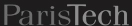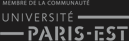Fermer X

# Risk and Decision

Michel De Lara
(last modiﬁcation date: October 10, 2017)
Version pdf de ce document
Version sans bandeaux

### Contents

We consider uncertainty modelled here by a set Ω, containing issues or scenarios. We present some classical measures attached to a scalar prospect (random variable) X : Ω . Here, X represents a gain, a proﬁt, a position, the value of a portfolio, etc., in a word a prospect while X represents a loss.

When focusing on situations of risk, we shall suppose that Ω carries a probability (deﬁned on a σ-ﬁeld , so that (Ω,, ) is a probability space). The expectation under probability is denoted by 𝔼.

### 1 Value at Risk

The Value at Risk of a prospect X at level λ ]0, 1[ is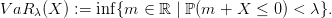(1)

Intuitively, saying that the V aR5% of a portfolio is 100 means that the loss X will be larger than 100 with probability at most 5%. However, V aR5% does not inform on the size of the loss.

#### 1.1 Value at Risk of a log-normal prospect

Question 1 Compute the Value at Risk at diﬀerent levels (1 %, 5 %, 10 %) of a log-normal prospect. Draw the empirical cumulated distribution of a log-normal prospect.

A prospect X is said to be log-normal if X > 0 and log X ∼𝒩(μ,σ2).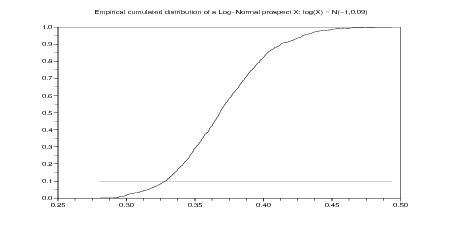Figure 1: Empirical cumulated distribution of a Log-Normal prospect X, such that log X 𝒩(μ,σ2) with μ = 1 and σ2 = 0.09. We observe that V aR 10%(X) ≈−0.33.

stacksize(2*10^8);
N=10^8; // number of simulations
mean=-1;
variance=0.09;
Y=grand(1,N,'nor',mean,variance) ; // simulation of a Normal r.v.
Z=sort(exp(Y));
// decreasing sort of the simulated realizations of a Log-Normal r.v.
values=  Z(\$:-1:1) // increasing sorting

// the VaR is -quantile
lambda=0.01;
quantile=values(int(lambda*N));
disp('VaR_('+string(lambda) +')(Z)='+string(-quantile))

lambda=0.05;
quantile=values(int(lambda*N));
disp('VaR_('+string(lambda) +')(Z)='+string(-quantile))

lambda=0.10; // level
quantile=values(int(lambda*N));
disp('VaR_('+string(lambda) +')(Z)='+string(-quantile))

xset('window',10) ; xbasc(); plot2d(values,linspace(0,1,N));
plot2d(values,lambda*ones(values),style=5);
xtitle('Empirical cumulated distribution of a Log-Normal prospect X: ...
log(X) ~ N(' +string(mean) +',' +string(variance)+ ')')

xset('window',11) ; xbasc(); histplot(10,Z);
xtitle('Empirical histogram of a Log-Normal prospect X: ...
log(X) ~ N(' +string(mean) +',' +string(variance)+ ')')

#### 1.2 Value at Risk and portfolio diversiﬁcation

Corporate bonds are sold at 95 \$ and return 100 \$ except in 2 % of cases where they lose all value. The prospect Xi provided is thus Xi = 100𝜀i 95 ∈{5,95}, where (𝜀i = 1) = 0.98 and (𝜀i = 0) = 0.02.

First, let us consider a fully concentrated portfolio C = 100X1, consisting of 100 units of the ﬁrst bond. Second, let us consider a fully diversiﬁed portfolio D = 2 i=150X i, consisting of 2 units of each bond. The 𝜀1, …, 𝜀50 are supposed to be independent.

Question 2 Using the fact that V aR5%(𝜀1) = 1, and positive homogeneity and translation-invariance of V aR5%, compute V aR5%(X1), then V aR5%(C). Estimate V aR5%(D) by simulation. Conclude that withdrawing up to 500 \$ from the fully concentrated portfolio C makes it acceptable, while you need to add a capital of at least 100 \$ to the fully diversiﬁed portfolio D to make this latter acceptable.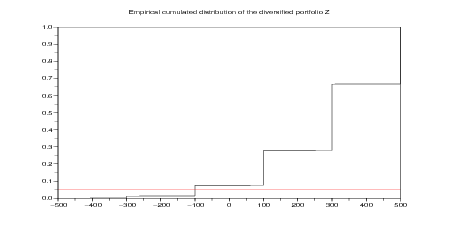Figure 2: Empirical cumulated distribution of the diversiﬁed portfolio

N=1000;
bb=50; // 50 independent couples of bonds
A=rand(bb,N);
EPS= 0.5 * ( 1 + sign(A-0.02) ) ;
// an array where each column contains
// bb independent realizations of the r.v. epsilon
DD=sum( 2 * (100 * EPS - 95 ) , 'r') ;
// N realizations of the diversified portfolio D

// xset('window',12) ; xbasc(); histplot(10,DD);
// xtitle('Empirical histogram of the diversified portfolio D')

SDD=sort(DD);
values=  SDD(\$:-1:1);
lambda=0.05;

xset('window',13) ; xbasc(); plot2d(values,linspace(0,1,N));
plot2d(values,lambda*ones(values),style=5);
xtitle('Empirical cumulated distribution ...
of the diversified portfolio DD')

VaR=-values(int(lambda*N));
disp('The Value at Risk of the diversified portfolio D is ')
disp('VaR_('+string(lambda) +')(DD)='+string(VaR))

### 2 Expected utility theory

The lottery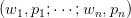describes a hazard situation where

• the outcome w1 may appear with probability p1,
• wn with probability pn,

where all probabilities are greater than or equal to zero and sum up to one (100% chance).

Suppose that the outcomes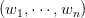are scalar. Let us be given a utility function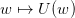. The value of the lottery is the expectation of the utilities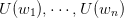: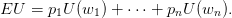In a choice situation between two lotteries, the expected utility maximizer will select the one with the highest EU.

#### 2.1 Expected utility of a simple lottery

3 \$ 50% -1 \$50%

Question 3 Compute the expected utility of the lottery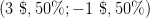for the utility function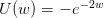.

function v=EU(lottery,utility)
values=utility(lottery(1,:));
probabilities=lottery(2,:);
v=sum(probabilities.*values) ;
endfunction

lottery=...
[3 -1; // outcomes
0.5 0.5] ; // probabilities

function u=utility(w)
u=-%e^{-2*w};
endfunction

v=EU(lottery,utility)

##### Experiment 1

Lottery 1Y 1 000 000 \$ 100% versus Lottery 1Z 1 000 000 \$ 89% 0 \$1% 5 000 000 \$10%

Question 4 Which of the two above lotteries do you personnaly prefer? Load the function EU following Question 3. Compute the expected utility of the lotteries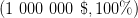and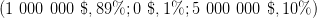for diﬀerent utility functions:

• CARA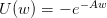, A > 0;
• CRRA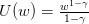, γ > 0, γ = 1, w > 0.

For each utility function, tell which lottery is prefered to the other one, and explain the reasons of your choice.

lottery_1Z=...
[1000000 0 5000000; // outcomes
0.89 0.01 0.1] ; // probabilities

A=1;
function u=CARA(w)
u=-%e^{-A*w};
endfunction

v_1Z=EU(lottery_1Z,CARA)

gama=0.5;
function u=CRRA(w)
u= w^{1-gama}/(1-gama) ;
endfunction

v_1Z=EU(lottery_1Z,CRRA)

##### Experiment 2

Lottery 2Y 1 000 000 \$ 11% 0 \$89% versus Lottery 2Z 5 000 000 \$ 10% 0 \$90%

Question 5 Same questions as in Question 5 for the lotteries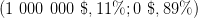and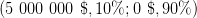.

Question 6 What do you conclude from your own preferences, compared to the expected utility evaluations of Questions 4 and 5? What is the relation with Allais’ paradox?

#### 2.3 Bank questionnaire

In a bank questionnaire, the customer answers a series of questions ranging from 8 to 13. In question k, he is asked which lottery he prefers between a certain lottery LOTTERY_C(k) and a 50%-50% risky lottery LOTTERY_R(k).

LOTTERY_C=list();
LOTTERY_R=list();

LOTTERY_C(8)=...
[20000; // outcomes
1] ; // probabilities

LOTTERY_R(8)=...
[40000 13400; // outcomes
0.5 0.5] ; // probabilities

LOTTERY_C(9)=...
[20000; // outcomes
1] ; // probabilities

LOTTERY_R(9)=...
[40000 10000; // outcomes
0.5 0.5] ; // probabilities

LOTTERY_C(10)=...
[20000; // outcomes
1] ; // probabilities

LOTTERY_R(10)=...
[40000 16000; // outcomes
0.5 0.5] ; // probabilities

LOTTERY_C(11)=...
[3000; // outcomes
1] ; // probabilities

LOTTERY_R(11)=...
[6000 2000; // outcomes
0.5 0.5] ; // probabilities

LOTTERY_C(12)=...
[3000; // outcomes
1] ; // probabilities

LOTTERY_R(12)=...
[6000 1500; // outcomes
0.5 0.5] ; // probabilities

LOTTERY_C(13)=...
[3000; // outcomes
1] ; // probabilities

LOTTERY_R(13)=...
[6000 2400; // outcomes
0.5 0.5] ; // probabilities

Question 7 Load the function EU following Question 3. Test wether lottery LOTTERY_C(k) is prefered to the 50%-50% risky lottery LOTTERY_R(k) for k = 8,, 13, for the CARA utility functions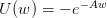, A > 0. Compute the Arrow-Pratt coeﬃcient of absolute risk aversion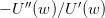. Select a proper range of values for the parameter A. How do you explain what you observe when the parameter A varies?

TEST_CARA=[];

for k=8:13
lottery_C=LOTTERY_C(k);
lottery_R=LOTTERY_R(k);
v_C=[];
v_R=[];

rangeA=10^(-4)*[0.3:0.5:4];

for A=rangeA
function u=CARA(w)
u=-%e^{-A*w};
endfunction
v_C=[v_C,EU(lottery_C,CARA)];
v_R=[v_R,EU(lottery_R,CARA)];
end

TEST_CARA=[TEST_CARA;v_C-v_R];
end

Question 8 Same question for the CRRA utility functions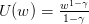, γ > 0, γ = 1, w > 0. Compute the Arrow-Pratt coeﬃcient of relative risk aversion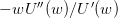. How do you explain what you observe when the parameter γ varies? Compare your results with the one in the document Lettre à S. by the economist Laurent Denant-Boemont. Do you agree with all his comments?

TEST_CRRA=[];

for k=8:13
lottery_C=LOTTERY_C(k);
lottery_R=LOTTERY_R(k);
v_C=[];
v_R=[];

//  for gama=[0.5 1.5] //
for gama=0.05:0.2:2 // to avoid gama=1
function u=CRRA(w)
u= w^{1-gama}/(1-gama) ;
endfunction
v_C=[v_C,EU(lottery_C,CRRA)];
v_R=[v_R,EU(lottery_R,CRRA)];
end

TEST_CRRA=[TEST_CRRA;v_C-v_R];
end

TEST_CRRA

Question 9 Same question for the power-expo utility functions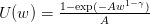, 1 > γ > 0, w > 0.

TEST_SAHA=[];

for k=8:13
lottery_C=LOTTERY_C(k);
lottery_R=LOTTERY_R(k);
v_C=[];
v_R=[];

A=0.08;
//  for gama=0.8
for gama=0.05:0.2:1
function u=SAHA(w)
u=(1-%e^{-A*w^(1-gama)})/A;
endfunction
v_C=[v_C,EU(lottery_C,SAHA)];
v_R=[v_R,EU(lottery_R,SAHA)];
end

TEST_SAHA=[TEST_SAHA; v_C-v_R ];
end

TEST_SAHA

#### 2.4 Test your own relative risk aversion

(from (Gollier2001, p. 30)).

Suppose that you are a decision-maker who evaluates any prospect X by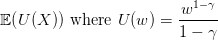(2)

is a CRRA (constant relative risk aversion) utility function. The parameter γ > 0 is the relative risk aversion parameter.

Consider the situation where you face the risk of gaining or losing a share 𝜃 of your wealth w0 with equal probability. The relative risk premium πγ(𝜃) is implicitely deﬁned by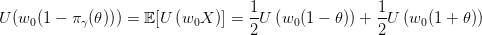(3)

where X takes values 1 𝜃 and 1 + 𝜃 with equal probability 12. The relative risk premium πγ(𝜃) is the share of your wealth that you are ready to pay to escape this risk.

Question 10 Give the formula for πγ(𝜃). For each 𝜃 =5 %, 15 %, 25 %, draw the curve giving the relative risk aversion parameter γ as a function of the relative risk premium. Fill in the second column of Table 2.4 with your relative risk premium and compute your relative risk aversion. Comment on what you observe.

//

theta=0.25 ;
gama=0.5:0.9:40; // avoid the value gama=1
0.5*(1+theta).^{1-gama}).^{1 ./(1-gama)};

xtitle('Relative risk aversion parameter as function of ...
relative risk premium when theta= '+string(theta))

//=========================================================
disp("=======================================");

// istring=0;

for theta=[0.05:0.10:0.25];
str="for theta="+string(theta)+", " ;
// istring=istring+1;
yourgama=[];
deff('y=f(gama)', 'y=1-(0.5*(1-theta).^{1-gama}+...
yourgama=[yourgama,fsolve(2,f)];
end
// mess(istring)=str+"the relative risk premium is ";
disp("corresponds the relative risk aversion parameter")
disp(yourgama)
end

//=========================================================
yourgama=[];
for j=1:(prod(size(theta)))
deff('y=f(gama)', 'y=1-(0.5*(1-theta(j)).^{1-gama}+...
yourgama=[yourgama,fsolve(2,f)];
end
endfunction

theta=[0.05:0.10:0.25];
disp("My likely relative risk aversion parameters are")

//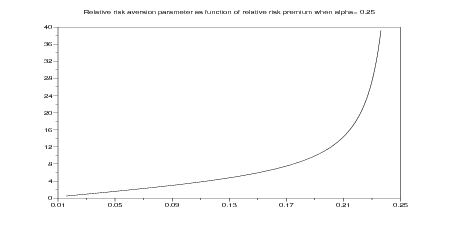Figure 3: Relative risk aversion parameter as function of relative risk premium for 𝜃 = 0.25

 gaining or losing fraction of your wealth relative risk aversion a share 𝜃 of your wealth that you are ready to pay parameter (empirical risk premium) 5 % 15 % 25 %

Table 1: Fill in the second column with your relative risk premium and compute your relative risk aversion. Check the range of parameters γ obtained.

### 3 Cumulative Prospect Theory

#### 3.1 Deﬁnition

(From (Kahneman and Tversky1979Tversky and Kahneman1992))

Suppose that the prospect X takes a ﬁnite number of values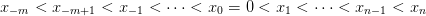(4)

with corresponding probabilities (nonnegative and summing up to one)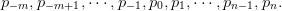(5)

The evaluation of a prospect with null anchor x0 = 0 is given by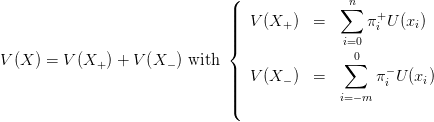(6)

where U is a strictly increasing function such that U(0) = 0. When the anchor x0 is not necessarily zero, we evaluate the prospect by V (X x0). The weighting functions for gains and losses are given by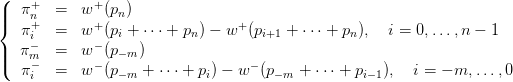(7)

where w+ and w are increasing functions satisfying w+(0) = w(0) = 0 and w+(1) = w(1) = 1. In (Tversky and Kahneman1992), one ﬁnds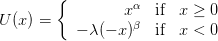(8)

with experimental evidence consistent with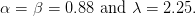(9)

For the weighting functions, (Tversky and Kahneman1992) proposes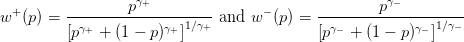(10)

with parameter values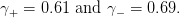(11)

#### 3.2 General Scilab code

//

function w=distorsion(p,ggamma)
w= p^ggamma ./ ( ( p^ggamma + (1-p)^ggamma )^{1/ggamma} )
endfunction

ggamma_plus= 0.61;
ggamma_minus= 0.69;

function pi=weight(proba,ggamma)
cumul_proba=cumsum(proba);
distort_cumul_proba = distorsion( cumul_proba , ggamma ) ;
ip = distort_cumul_proba - [0 distort_cumul_proba(1:(\$-1))];
pi=ip(\$:(-1):1);
endfunction

alpha=0.88;
bbeta=0.88;
lambda=2.25;

function [H] = Heavyside(x)
// Heavyside function
H = bool2s(x>=0)
// notice that Heavyside(0)=1
endfunction

function u=utility(x)
u=Heavyside(x).*x^alpha - lambda* Heavyside(-x).* (-x)^bbeta
endfunction

function v=CPT_eval(lotery,anchor)
values=lotery(1,:)-anchor;
probabilities=lotery(2,:);
//
ind_plus=find(values > 0) ;
values_plus=values(values > 0) ;
proba_plus = probabilities(ind_plus);
aborp_plus=proba_plus(\$:(-1):1);
utility_plus=utility(values_plus) ;
weighting_plus= weight ( aborp_plus , ggamma_plus) ;
//
ind_minus=find(values <= 0) ;
values_minus=values(values <= 0) ;
proba_minus = probabilities(ind_minus);
utility_minus=utility(values_minus) ;
weighting_minus= weight ( proba_minus , ggamma_minus) ;
//
v= sum(utility_plus .* weighting_plus) + ...
sum(utility_minus .* weighting_minus)
endfunction

//

Question 11 Same questions as in §2.2, but evaluating lotteries by CPT, with either zero anchor, or a positive one that you will choose.

anchor=0;
v=CPT_eval(lottery_1Z,anchor)

#### 3.4 Bank questionnaire

Question 12 Same questions as in §3.4, but evaluating lotteries by CPT, with either zero anchor, or the value of the certain lottery.

v_C=[];
v_R=[];

for k=8:13
lottery_C=LOTTERY_C(k);
lottery_R=LOTTERY_R(k);
//
anchor=0;
anchor=lottery_C(1,:);
//
v_C=[v_C,CPT_eval(lottery_C,anchor)];
v_R=[v_R,CPT_eval(lottery_R,anchor)];
end

TEST_CPT=v_C-v_R

#### 3.5 Relative risk aversion

Let us consider the problem in Sect. 2. Suppose that you face the risk of gaining or losing a share 𝜃 of your wealth x0 with equal probability. The relative risk premium πCPT (𝜃) is the share of your wealth that you are ready to pay to escape this risk. Expressing indiﬀerence between this risk and the certain prospect x01 𝜃gives: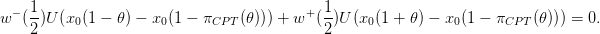(12)

Notice that (8) with α = β implies that the evaluation of a prospect with respect to an anchor is invariant with respect to a positive scaling factor (of both the prospect and the anchor). We thus write the above equation with x0 = 1, to obtain: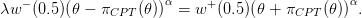(13)

In the end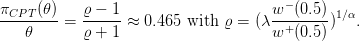(14)

rho = ( lambda * weight(0.5,ggamma_minus) / ...
weight(0.5,ggamma_plus) )^{1/alpha}

factor= (rho-1)/(rho+1)

 gaining or losing a share 𝜃 of your wealth CPT relative risk premium 5 % 2.3 % 15 % 7 % 25 % 11.6 %

Table 2: Relative risk premium evaluated by Cumulative Prospect Theory (CPT)

#### 3.6 Compte d’épargne MMmax by Mutuelles du Mans

(From (Pﬁﬀelmann and Roger2005))

The compte d’épargne MMmax was launched in November 2003 by Mutuelles du Mans. This ﬁnancial product returns 2.5 % for sure every year and, when lucky, additional 5 %, 10 % or 20 % (see details in the Scilab code below). We want to test its success to the public by comparison with a safe product returning a ﬁxed rate every year (anchor).

Question 13 The values for MMmax are given below. Show that its mean return is slightly more than 3.5% Draw the evaluation of this product as function of the anchor for an investor evaluating prospects according to Cumulative Prospect Theory (CPT investor, in short). Observe that any safe product with return more than 3.3% is prefered to the risky MMmax. Conclude that a CPT investor is less encline to invest in MMmax than in safe product, compared to a risk-neutral investor.

//

lotery_MMmax=[1025 1075 1125 1225 ; // return for 1000 euros
0.81 0.171 0.0171 0.0019] ; // probabilities
// Compte d'\'epargne MMmax launched in November 2003 by
// Mutuelles du Mans

disp("=======================================");
disp("This is the MMmax lotery for an investment of 1000 euros")
disp(lotery_MMmax)

//=========================================================

function v=scaling(w)
v=(w-1000)/10; // converts from euros to percents
endfunction

function w=unscaling(v)
w=1000 + 10*v ; // converts from percents to euros
endfunction

function v=RN_eval(lotery,anchor)
// Risk Neutral evaluation in euros
v= sum(prod(lotery,'r')) - anchor ;
endfunction

//=========================================================

exec CPT.sci

mean_MMmax= RN_eval(lotery_MMmax,0) ;
mean_MMmax_percent=scaling(mean_MMmax);

disp("=======================================");
disp('The expected return of the MMmax lotery is '...
+string(mean_MMmax_percent) +'%');

//=========================================================

step=0.01 ;
anchors_percent=[3.0 : step : (mean_MMmax_percent+20*step) ];
anchors=unscaling(anchors_percent) ; // return for 1000 euros
CPT_Values=[];RN_Values=[];

// getf('CPT.sci')

for anchor=anchors
CPT_Values=[CPT_Values,CPT_eval(lotery_MMmax,anchor)];
RN_Values=[RN_Values,RN_eval(lotery_MMmax,anchor)];
end

xset('window',30)
xbasc(); plot2d(anchors_percent,...
[ 0*ones(anchors) ; CPT_Values ; RN_Values ]' );
xtitle('CPT and RN evaluation of MMmax ...
as function of the anchor','anchor (%)','')
legends([ 'Cumulative Prospect Theory (CPT)'; 'Risk Neutral (RN)' ] ,...
[2,3], 'ur' );
xstring(2.95,-1.5,["RN and CPT prefer" ; "MMax to safe"],0,0)
xstring(3.3,1.5,["RN prefers MMax to safe but not CPT"],30,1)
xstring(3.55,0.5,["RN and CPT prefer" ; "safe to MMax"],0,0)

//=========================================================

anchor=1033.37;
disp("for annual safe return anchor of " +string(scaling(anchor)) +"%, ...
the CPT evaluation is " +string(CPT_eval(lotery_MMmax,anchor)))

anchor=1033.38;
disp("for annual safe return anchor of " +string(scaling(anchor)) +"%, ...
the CPT evaluation is " +string(CPT_eval(lotery_MMmax,anchor)))

//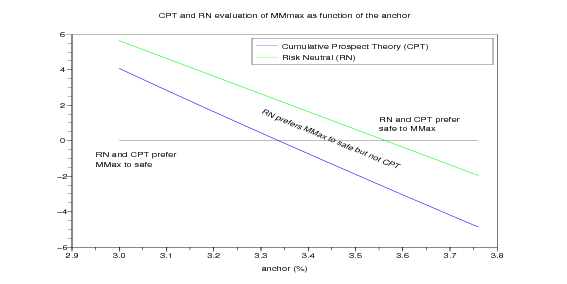Figure 4: Evaluation of MMmax as function of the anchor. When the evaluation is positive, an investor prefers MMmax to the safe return in abscisse. A risk neutral investor prefers MMmax to any safe return between 3.338% and 3.564%, while this is the contrary for a CPT investor.

### References

Rudiger Frey and Alexander J. McNeil. Var and expected shortfall in portfolios of dependent credit risks: Conceptual and practical insights. Journal of Banking and Finance, 26:1317–1334, 2002.

C. Gollier. The Economics of Risk and Time. MIT Press, Cambridge, 2001.

Daniel Kahneman and Amos Tversky. Prospect theory: An analysis of decision under risk. Econometrica, 47(2):263–292, 1979.

Marie Pﬁﬀelmann and Patrick Roger. Les comptes d’épargne associés à des loteries: approche comportementale et étude de cas. Banque & Marchés, septembre 2005.

Amos Tversky and Daniel Kahneman. Advances in prospect theory: Cumulative representation of uncertainty. Journal of Risk and Uncertainty, 5(4):297–323, October 1992.

 L'École des Ponts ParisTech est membre fondateur de L'École des Ponts ParisTech est certifiée